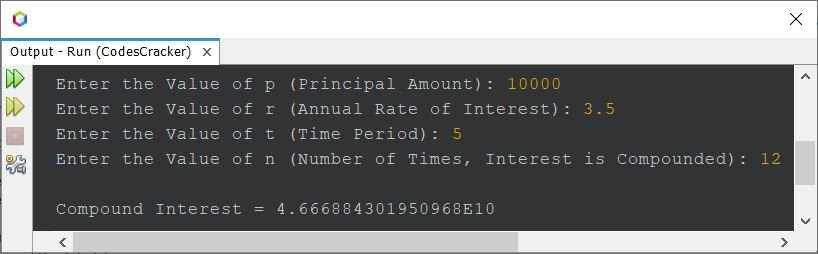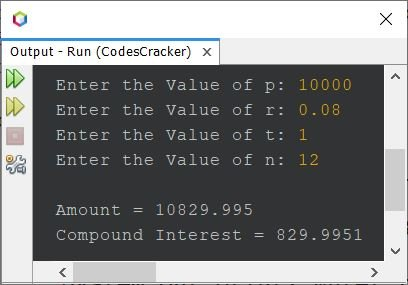# Java Program to Calculate Compound Interest

The formula to find compound interest is:

`CI = (1 + r/n)(nt) - p`

where CI indicates compound interest, p indicates principal amount, r indicates the annual rate of interest, t is the time period for which the money is invested or borrowed, and n indicates the number of times that the interest is compounded per unit t.

Note: If the interest is compounded monthly, then the value of n would be 12. If the interest is compounded quarterly, then the value of n would be 4, and so on. This happens when t is in years.

The question is: write a Java program to find and print the compound interest based on the data such as p, r, t, and n values. The data must be received by the user at the runtime of the program. The program given below has its answer:

```import java.util.Scanner;

public class CodesCracker
{
public static void main(String[] args)
{
double p, r, t, n, ci;
Scanner s = new Scanner(System.in);

System.out.print("Enter the Value of p (Principal Amount): ");
p = s.nextDouble();
System.out.print("Enter the Value of r (Annual Rate of Interest): ");
r = s.nextDouble();
System.out.print("Enter the Value of t (Time Period): ");
t = s.nextDouble();
System.out.print("Enter the Value of n (Number of Times, Interest is Compounded): ");
n = s.nextDouble();

ci = p * Math.pow(1 + (r/n), (n*t)) - p;

System.out.println("\nCompound Interest = " +ci);
}
}```

The snapshot given below shows the sample run of the above Java program with user inputs of 10000 as the principal amount, 3.5 as the rate of interest, 5 as the time period, and 12 as the number of times that the interest is compounded.The above program can also be created in this way. This program prints the value of the amount too.

```import java.util.Scanner;

public class CodesCracker
{
public static void main(String[] args)
{
Scanner s = new Scanner(System.in);

System.out.print("Enter the Value of p: ");
float p = s.nextFloat();
System.out.print("Enter the Value of r: ");
float r = s.nextFloat();
System.out.print("Enter the Value of t: ");
float t = s.nextFloat();
System.out.print("Enter the Value of n: ");
float n = s.nextFloat();

float amount = p * (float)Math.pow(1 + (r/n), (n*t));
float ci = amount - p;

System.out.println("\nAmount = " +amount);
System.out.println("Compound Interest = " +ci);
}
}```

Here is its sample run with user input 10000 as p, 0.08 as r, 1 as t, and 12 as n:Java Online Test

« Previous Program Next Program »